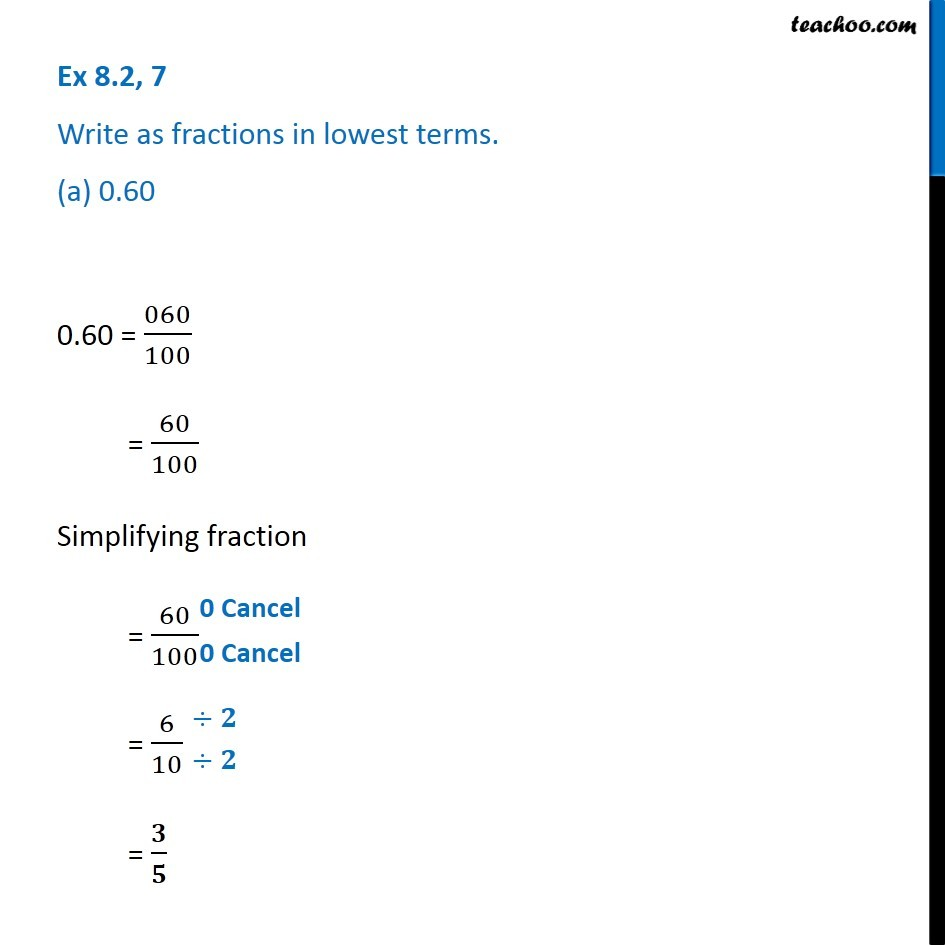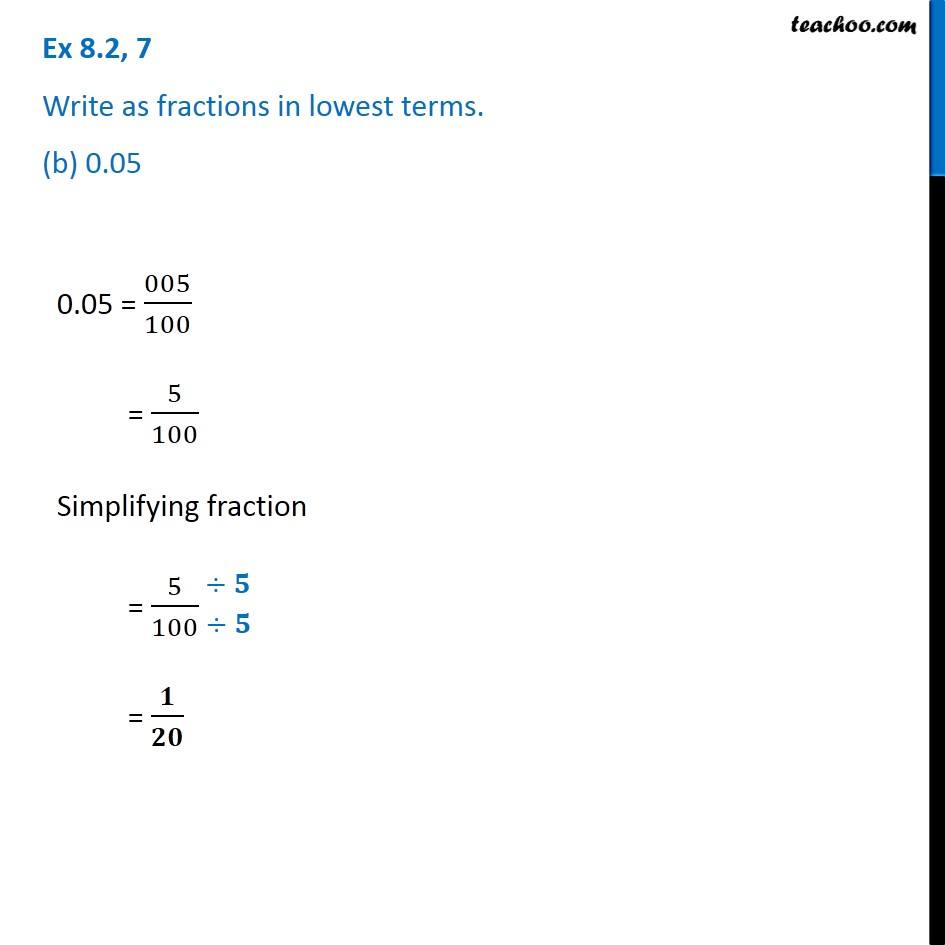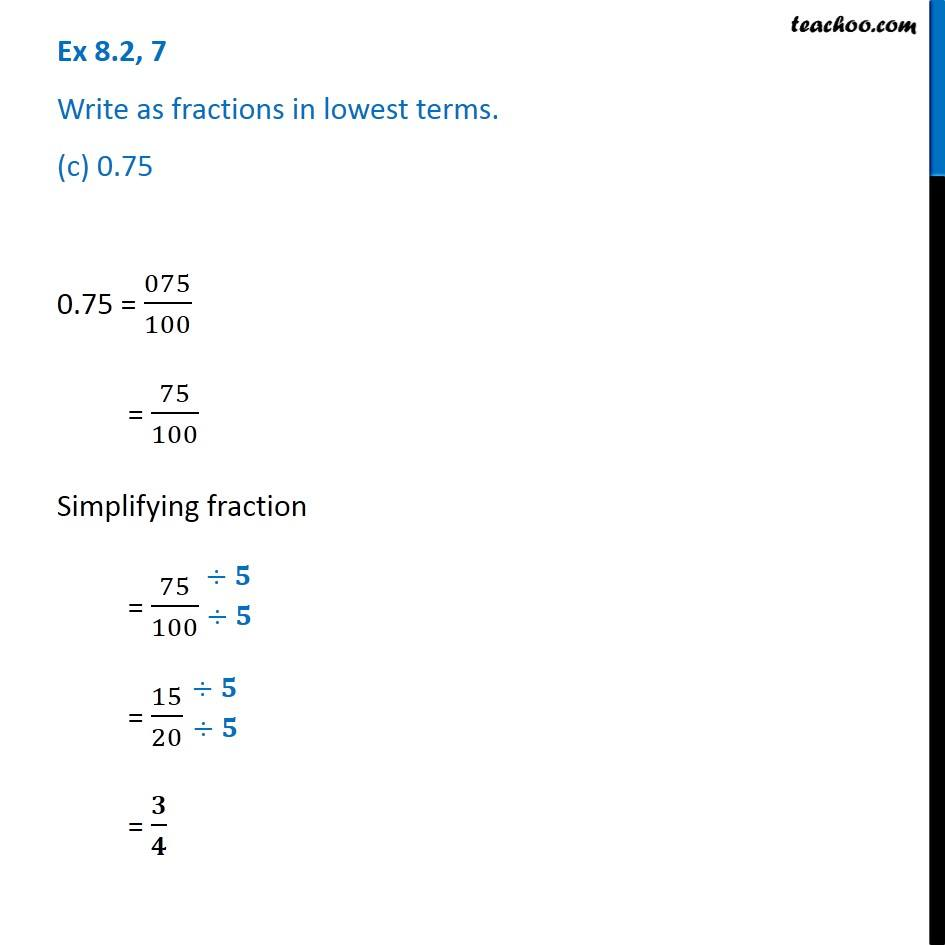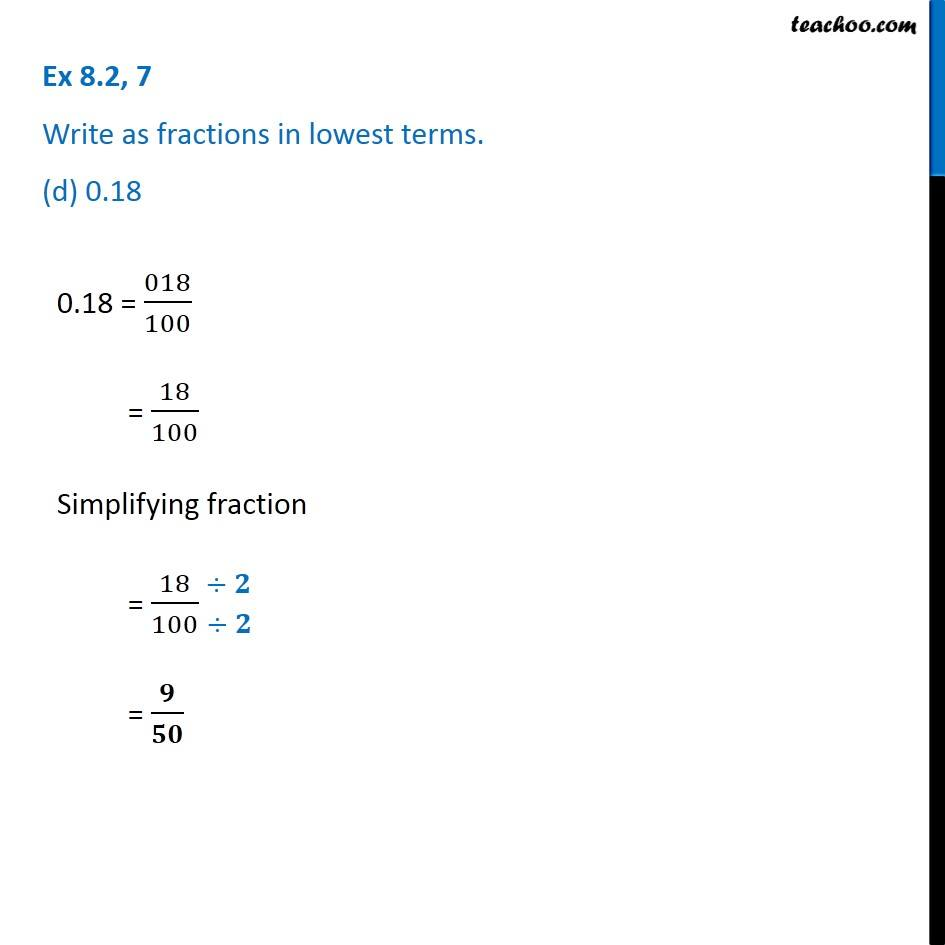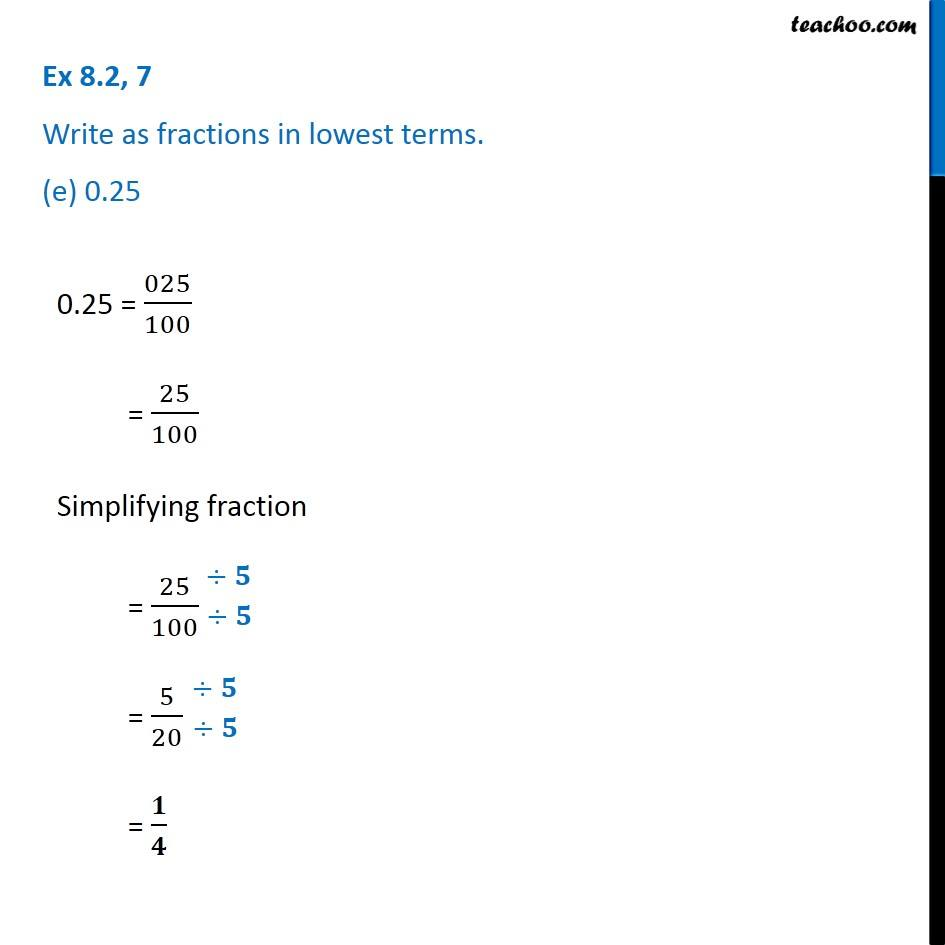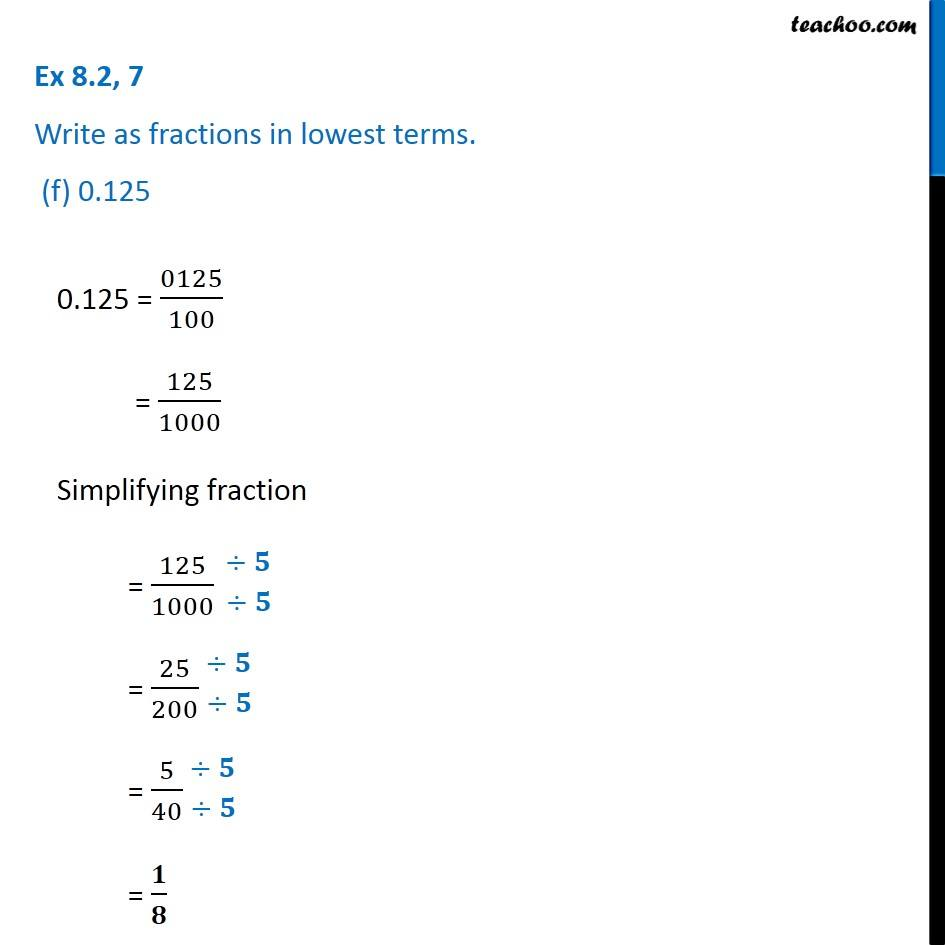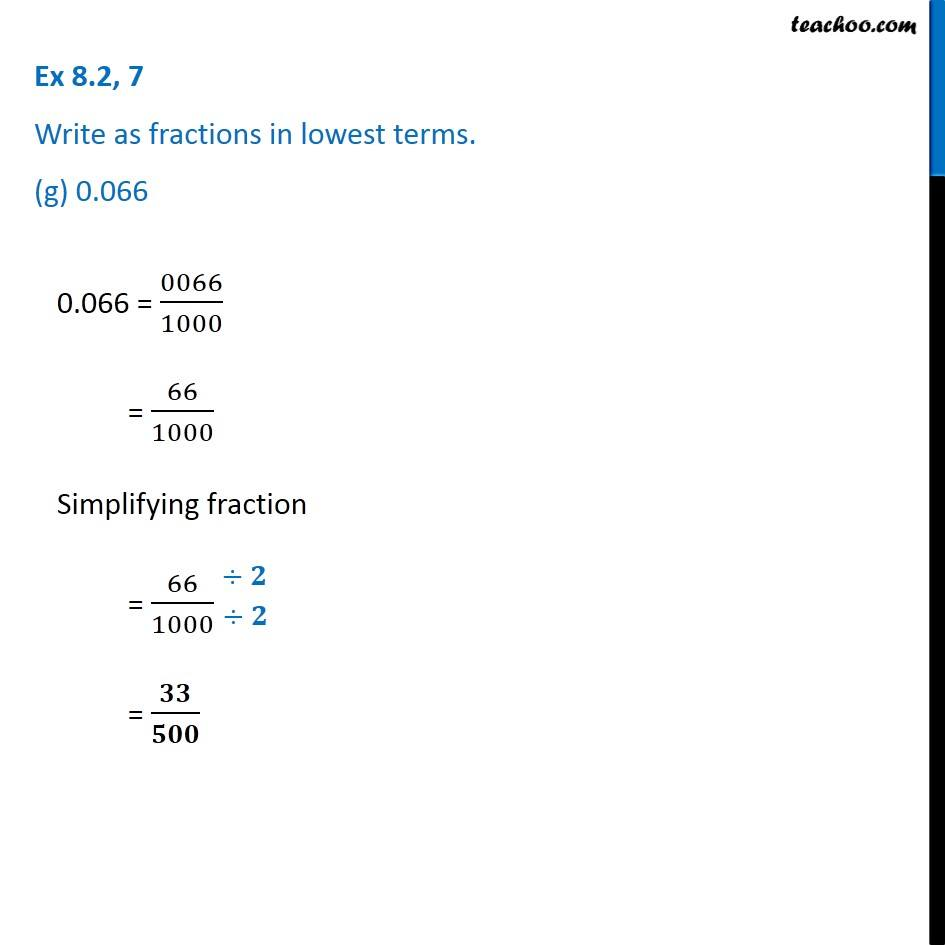Subscribe to our Youtube Channel - https://you.tube/teachoo

1. Chapter 8 Class 6 Decimals
2. Serial order wise
3. Ex 8.2

Transcript

Ex 8.2, 7 Write as fractions in lowest terms. (a) 0.60 0.60 = 060/100 = 60/100 Simplifying fraction = 60/100 = 6/10 = 𝟑/𝟓 Ex 8.2, 7 Write as fractions in lowest terms. (b) 0.05 0.05 = 005/100 = 5/100 Simplifying fraction = 5/100 = 𝟏/𝟐𝟎 Ex 8.2, 7 Write as fractions in lowest terms. (c) 0.75 0.75 = 075/100 = 75/100 Simplifying fraction = 75/100 = 15/20 = 𝟑/𝟒 Ex 8.2, 7 Write as fractions in lowest terms. (d) 0.18 0.18 = 018/100 = 18/100 Simplifying fraction = 18/100 = 𝟗/𝟓𝟎 Ex 8.2, 7 Write as fractions in lowest terms. (e) 0.25 0.25 = 025/100 = 25/100 Simplifying fraction = 25/100 = 5/20 = 𝟏/𝟒 Ex 8.2, 7 Write as fractions in lowest terms. (f) 0.125 0.125 = 0125/100 = 125/1000 Simplifying fraction = 125/1000 = 25/200 = 5/40 = 𝟏/𝟖

Ex 8.2

Chapter 8 Class 6 Decimals
Serial order wise